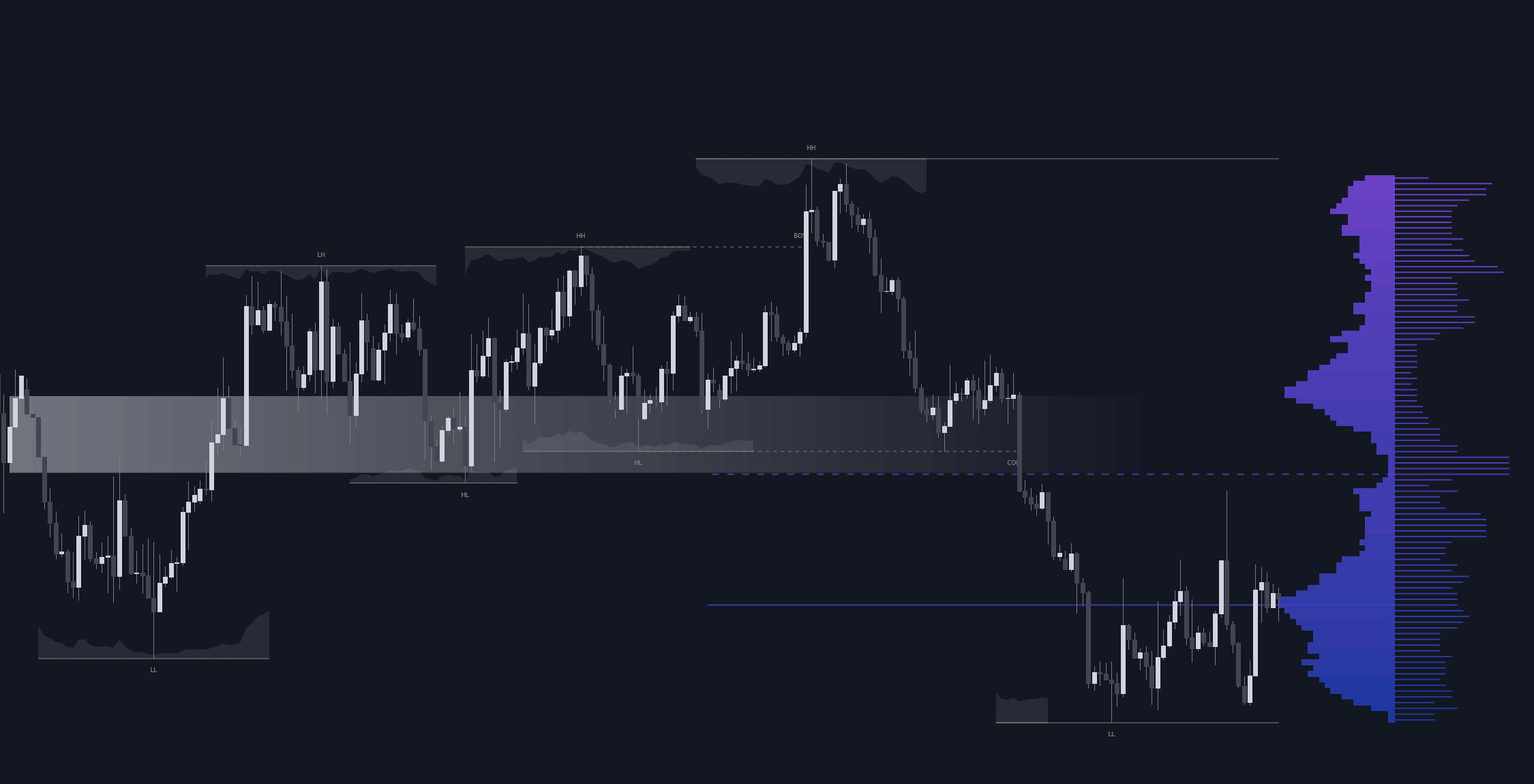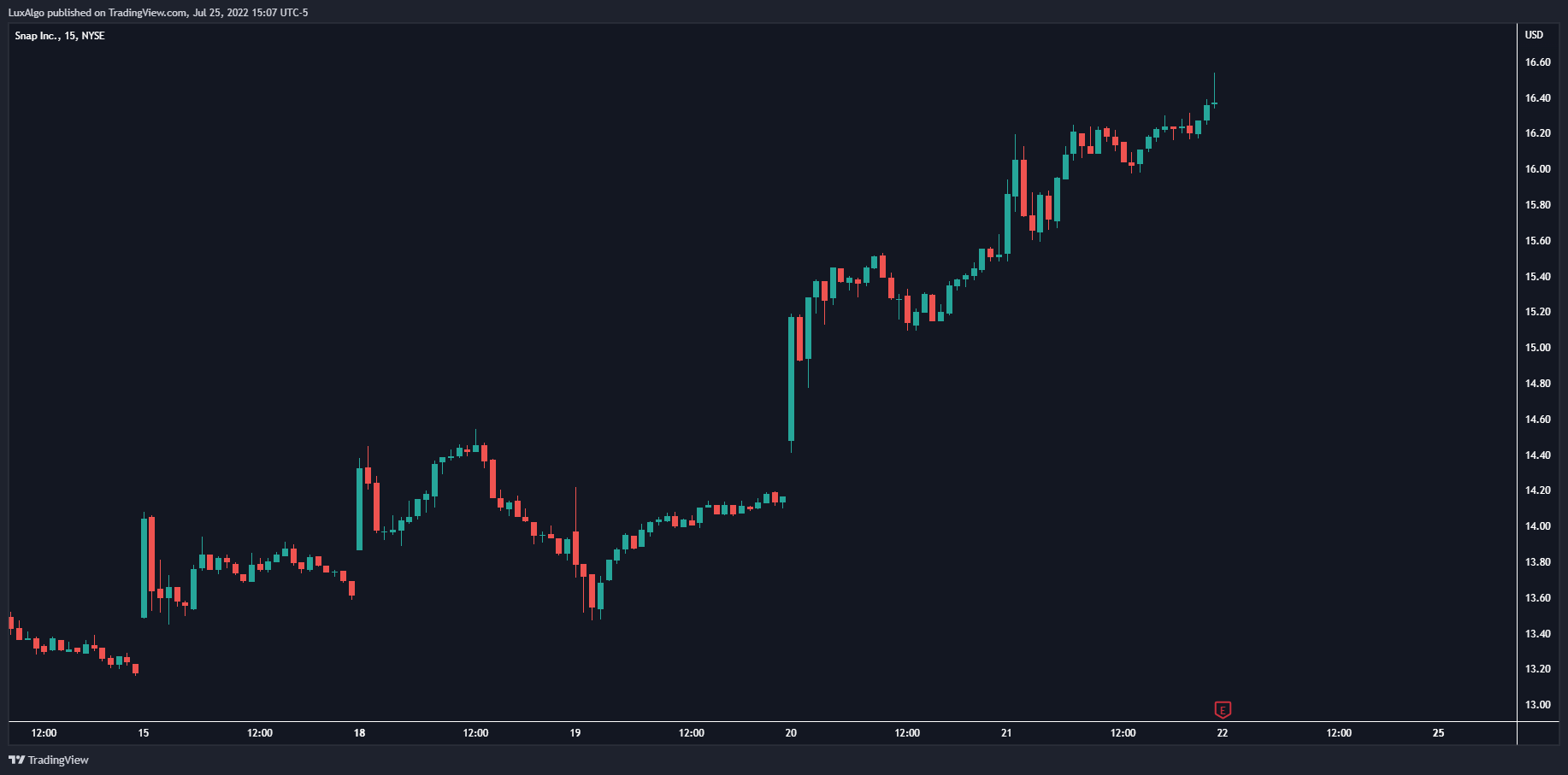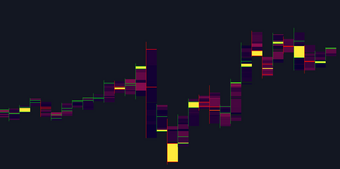# A Deep Dive Into The Relative Strength Index

Oscillators play an important role in technical analysis due to the amount of information they can return as well as their ability to lead price variations and are often used in conjunction with trend indicators. This post offers a deep dive into this classic oscillator, sharing observations as well as reviewing derived indicators.

Oscillators play an important role in technical analysis due to the amount of information they can return as well as their ability to lead price variations and are often used in conjunction with trend indicators.

One of the most popular oscillators available is the relative strength index ( RSI ), one of the many popular indicators developed by J. Welles Wilder and first appearing in "New Concepts in Trading Systems".

This post offers a deep dive into this classic oscillator, sharing observations as well as reviewing derived indicators.

### Introduction

The relative strength index is a normalized (bounded) oscillator in the range (0,100) and aims to indicate when the price is overbought (that is when a security is being traded above its intrinsic value) or oversold (that is when a security is being traded under its intrinsic value) and when a reversal might occur.

In order to determine when the security is overbought or oversold the RSI includes one constant overbought (70 suggested by Wilder) and one oversold level (30 suggested by Wilder). An RSI value above the overbought level indicates that the security is overbought, while an RSI value under the oversold level indicates that the security is oversold.

The RSI possesses one length user setting allowing the user to obtain information regarding shorter or longer-term price variations, often set as 14 by default. This default setting value is often unchanged.

### Calculation

The various calculations for the RSI reveal much interesting information regarding its construction as well as the information the indicator aims to give.

The RSI is generally calculated as follows:

delta = close - close
upward = max(delta,0)
downward = max(-delta,0)
relative_strength = rma(upward,length)/rma(downward,length)
relative_strength_index = 100 - 100/(1 + relative_strength)

where close is the closing price, relative_strength the relative strength , and rma the Wilders moving average (RMA), sometimes referred to as smoothed moving average ( SMMA ), which is an exponential filter using the smoothing constant 1/length instead of 2/(length+1) in the case of an exponential moving average .

A more approachable calculation for the RSI is given by:

RSI = rma(upward,length)/rma(abs(delta),length) × 100

#### Choice Of Moving Average For The RSI Calculation

Choosing which moving average to use for the calculation of the RSI can affect the results returned by the indicator, as well as the way to calculate it.

The original calculation makes use of the Wilders moving average, but various authors make use of the simple moving average or exponential moving average.

#### Using The SMA

Cutler's version of the RSI makes use of the simple moving average for the calculation of the RSI and introduces the most notable changes compared to the EMA and Wilders moving average and has several properties.

First the calculation can be obtained with:

cutlers = (close - close[length])/length/MA(abs(delta),length) × 50 + 50

which further simplifies to:

cutlers = (close - close[length])/SUM(abs(delta),length) × 50 + 50

The SMA is more reactive than the RMA, which returns an RSI more prone to cross the overbought/oversold level compared to an RMA-based RSI of the same setting.

Additionally, a new input discards the oldest input in the calculation window of the SMA . This can introduce sharp changes in the RSI compared to one based on the EMA /RMA who retain memory of oldest inputs.

A downside of using the SMA is the potential occurrence of division by zero, altho unlikely it can still happen if the price of a security is equal to its previous value for a period superior to the used length setting, this would return a change in the price of 0, which ultimately could lead the denominator of the previous calculation to be equal to 0. This do not directly happen when using exponential filters for the RSI calculation.

#### Using The EMA

Unlike the SMA , using an exponential moving average does not introduce any particular significant changes. An RSI based on the EMA is more reactive than one based on the RMA when using the same length setting. However both the EMA and RMA are based on exponential averaging, only the smoothing constant differ, as such in order to obtain the length setting used in an RSI based EMA such that it is equal to an RMA based RSI we must solve the following equation with respect to len_ema:

1/len_rma = 2/(len_ema+1)
= 2 × len_rma - 1

As such a length 27 EMA-based RSI is equal to a length 14 RMA-based RSI .

### Effect Of The Length Setting On The RSI

When applying the RSI to certain securities with a large sample size, using a higher length setting would return results closer to the central value 50, thus returning a narrower RSI range.

In the image above, on the left, you can see the frequency histograms (as a line plot) using a bin size of 15 from various RSI outputs containing 500 observations using an increasing length setting. RSI's with higher length settings return a narrower shape, all having a peak value near 50. On the right, you can see the range of the RSI outputs value over the length setting (from 10 to 100), the range is decaying non-linearly.

This behavior is a frequent occurrence if the RSI indicator is applied to price changes having an approximately symmetrical distribution with 0 mean, which is often the case.

As such using higher length settings would return an RSI reaching less frequently its overbought/oversold level. This particularity differentiates the RSI from oscillators such as the stochastic oscillator, whose observed range does not change when using different length settings and makes the RSI particularly appropriate when it comes to identifying potential reversals.

### Interpretations

Wilder describes various ways to interpret the RSI .

#### Using Levels

The main one is given by the interaction between the RSI and the overbought and oversold levels, with an RSI value over the overbought or under the oversold level indicating a potential reversal or retracement. This sign of a reversal can be given by the RSI crossing over the overbought level and crossing under the oversold, this allows to obtain an early reversal detection but can expose the trader to a continuation of the trend. An RSI value crossing under the overbought level and crossing over the oversold level allows the trader to be less exposed to possible trend continuations but has a worse timing.

A more trend-following approach can be used, a value of the RSI superior to 50 would indicate an uptrend and a value under 50 would indicate a downtrend. While this approach provides a worse timing and can produce whipsaw trades, it allows the trader to follow the trend instead of going against it.

#### Using Patterns

Wilder also shows that classical chart patterns such as triangles, wedges , head & shoulders ...etc, and supports/resistances can be found and used within the RSI . These visible formations are the result of the detrended price observations given by the RSI , and as such can be more frequent. However, the noisy nature of the RSI might make the detection of these formations more complicated.

#### Using Divergences

Like with most oscillators, divergences between the RSI and the price can be used to detect potential reversals. A divergence occurs when the price tops/bottoms and RSI tops/bottoms are negatively correlated.

#### Using Failure Swings

Failure swings are characterized by the RSI making a significant high/low above the overbought/under the oversold level followed by a retracement which is then followed by a failed attempt of the RSI to reach the overbought/oversold level. The failure point triggers the trade and is determined by the maximum/minimum of the retracement following the high/low made by the RSI .

#### Connor's Period 2 RSI

Connor's Period 2 RSI is a short-term contrarian strategy developed by Larry Connors offering a different interpretation of the RSI indicator. As its name indicates the strategy is based on a period 2 RSI and mostly aims to trade retracements and potential reversals. The strategy makes uses a period 200 moving average to determine the overall price trends, a period 5 moving average for exits, and the period 2 RSI for entries.

A buy order is opened when the RSI crosses under the oversold level (set at 5) while the price is under the period 200 SMA , a sell order is opened when the RSI crosses over the overbought level (set at 95) while the price is above the period 200 SMA . A position is closed when the price crosses the period 5 SMA .

Using such a strategy would require using high timeframes to avoid frictional costs due to the short potential gains obtained from it.

### Other Indicators Using The RSI

The RSI is used by a wide variety of indicators, here are a few of them.

#### Stochastic RSI

The Stochastic RSI is as its name indicates a stochastic oscillator using the RSI as input. Since the RSI leads price variations this allows the Stochastic RSI to obtain an increased reactivity. The nature of the Stochastic oscillator calculation allows the Stochastic RSI to be in a range (0,100), which unlike the RSI does not converge toward 50 with a higher length of the RSI / Stochastic .

#### Ehlers Inverse Fisher Transform

In an article, Ehlers applies the inverse fisher transform (hyperbolic tangent function) to a smoothed RSI ( WMA with period 9), rescaled in the range (-5,5). As seen before the RSI tend to have a symmetrical distribution centered near 50, the inverse fisher transform applied to it allows obtaining a U-shaped distribution, in other words, it "squash" the original smoothed RSI , returning a result in a scale (-1,1).

#### Laguerre RSI

Another version of the RSI is presented by Ehlers in his article Time Warp – Without Space Travel.

This indicator does not make use of the RSI directly but instead consists of using multistage filters whose degree of smoothness is determined by a user setting gamma in a range (0,1), with values of gamma closer to 1 returning longer-term indications. For its calculation, the indicator calculates its numerator as the sum of the difference between one filter and the one following in the stage, and its denominator is the sum of the absolute difference between one filter and the one following in the stage until finally rescaling the ratio between the numerator and denominator in a (0,100) range that is:

L0 = (1 - gamma) × close + gamma × L0
L1 = -gamma × L0 + L0 + gamma × L1
L2 = -gamma × L1 + L1 + gamma × L2
L3 = -gamma × L2 + L2 + gamma × L3
//----
num = (L0 - L1) + (L1 - L2) + (L2 - L3)
den = abs(L0 - L1) + abs(L1 - L2) + abs(L2 - L3)
lrsi = 50*num/den + 50

Unlike a regular RSI , the Laguerre RSI does not converge near 50 with higher gamma values, having an approximately U-shaped distribution.

## Recent posts### Smart Money Concepts (SMC) Explained for Trading Forex, Stocks, & Crypto

##### September 9, 2022### The Best Time Frame for Trading (Actual Truth)

##### August 27, 2022### Liquidity Heatmap LTF

##### May 31, 2022